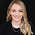### Teaching Elapsed Time on a Number Line

Are your students struggling with ELAPSED TIME
math problems? These strategies really helped my students!

Elapsed time is such a tricky concept to teach in a way that students understand. The passage of time is such an abstract concept that students really struggle to master this skill. There are a variety of methods and strategies for teaching elapsed time, but using a number line is one of my favorites. My students especially love using different colored pencils for each of the steps!

## Teaching Elapsed Time Using a Number Line - Strategy #1:

This first example is a very traditional way of teaching elapsed time using a number line, but with a unique twist.  To begin, students draw a straight line across the page, then label the left side with the starting time and the right side with the ending time. Next, we draw a vertical hash to represent the next hour after the start time. In this case, because the start time is 8:43 AM, the next hour will be 9:00 AM. I encourage students to use one color of pencil to jump above the number line in increments that make sense to them up to the next hour. In this case, we jumped 2 minutes to 8:45 AM, then 15 min to 9:00 AM, for a total of 17 minutes.

This is where I step aside from the super traditional route and have them move to the end of the number line. Now we move backwards and draw a vertical hash at the closest hour to the end time.
In this case, because the end time is 11:18 AM, the closest hour would be 11:00 AM. I encourage students to use one color of pencil to jump above the number line in increments back to the hour. It's really easy to label this one because it's always represented by the last two digits (or minutes) of the end time.

To finish up the number line, we jump below the number line from the two middle hash marks. In this case, we jump from 9:00 AM to 11:00 AM, which is a total of 2 hours. I like to have them mark this below the number line so that they see this number represents hours instead of minutes.

Now it's time to determine the total amount of elapsed time. My students really respond to using a T-Chart for this with the hours on the left and the minutes on the right. When we are in the beginning stages of learning this strategy, I have students use their matching colored pencils to record the numbers. This helps them visual how the T-Chart connects to their work on the number line. In the event that the minutes add up to 60 or greater, we use the traditional subtraction method to take away 60 minutes from the right and add 1 hour to the left. (This example didn't require it.)

The next example shows a longer period of elapsed time. These types of problems are especially tricky for my students. We follow the exact same steps as above. However, when they are determining the hours, I like to have them make a vertical hash exactly in the middle to represent 12 noon (or 12 midnight).  This helps prevent students from mistakenly subtracting the PM from the AM and getting the wrong amount of hours.

Again, we use the T-chart to determine the total amount of elapsed time by writing the hours on the left and the minutes on the right. Because the minutes add up to more than 60 in this example, we use the traditional subtraction method to take away 60 minutes from the right and add 1 hour to the left. (Be sure to read how much easier this problem is to solve when using strategy #2 below!)

## Teaching Elapsed Time Using a Number Line - Strategy #2

This next strategy is similar to using an analog clock to teach elapsed time, but it is represented on the number line. Honestly, my students and I find this strategy MUCH EASIER than the traditional number line strategy.

In this strategy, students begin by drawing a straight line across the page, then labeling the left side with the starting time and the right side with the ending time. Next, we draw a vertical hash to represent exactly one hour after the start time. In this case, because the start time is 8:43 AM, exactly one hour later will be 9:43 AM. We keep drawing vertical hashes until it is closest to the end time without going over.  In this example, we jumped 2 times below the number line to represent 2 hours that have passed.

We continue by making vertical hashes in minute increments that make the most sense to the students. This may vary depending on the child. In this example, we jumped 2 minutes from 10:43 AM to 10:45 AM, then 15 minutes to 11:00 AM, then the last 18 minutes to 11:18 AM.

Now we can determine the total amount of elapsed time by using a T-chart. We record the hours on the left and the minutes on the right, then add them up for their total. I think the students like this strategy even more than the first because they just move from left to right across the number line in increments that are really easy to determine.

Below is an example with a longer amount of elapsed time. While this problem was much trickier to solve using strategy #1, it's so much simpler with strategy #2!

I hope you have found these strategy explanations helpful to both you and your students. Let me know which strategy works best for you all in the comments below!

#### 1 comment

1.Thank you so much! I've just used this in class and it was fantastic. Thank you, thank you, thank you!!